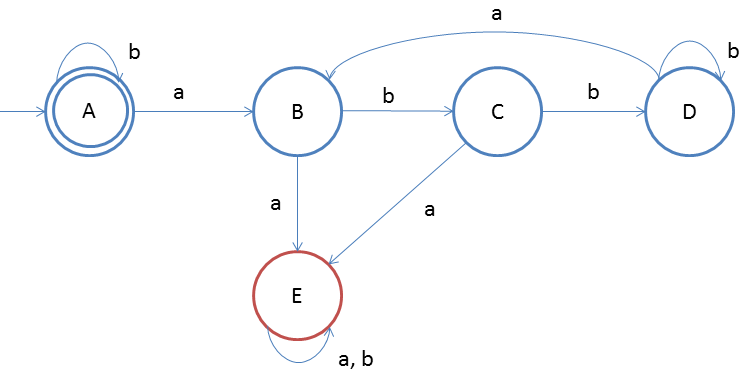# DFA String Examples

Design a DFA in which every 'a' should followed by 'bb'
Given: Input alphabet, Σ={a, b}
Language L = {ε, abb, abbabb, abbabbabb, babb, ...}

Clearly the language is infinite because there is infinite number of strings.The idea behind this approach is very simple, follow the steps below and you will understand.

1. Firstly there is ε so make start state as final state
2. And we do not care if string is only 'bbbbb...'(as this doesn't violate given property) so put self-loop on start state itself
3. if a comes then we will move state B and we should have two b's after that so state C and D(final state)
4. Now what if 'a' comes on state D so we will direct it to state B so that if two b's comes thereafter then string will be accepted
5. On state D if b comes then that is not a problem so a self-loop is given there for 'b'
6. What if 'a' comes on state B then it should be rejected as after 'a' only two b's are allowed
7. That is why we have a dead state E(in red)
8. On state C if 'a' comes then also property is violated so we will direct flow to state D on 'a'
9. On state E we do not care about either 'a' or 'b' as the stirng is already failed the property

### Testing

1. Lets take one input string babb
2. Scan string from left to right
3. First input is b, so from state A we will go to state A itself
4. Second input is a, so from state A we will go to state B
5. Third input is b, so from state B we will go to state C
6. Fourth input is b, so from state C we will go ti state D(final state)
After end of the string we are at final state so string is accepted.
You may also try some examples of strings to run over this DFA, and you will understand more